Next: Choosing the subset of Up: Link analysis Previous: Topic-specific PageRank   Contents   Index

# Hubs and Authorities

We now develop a scheme in which, given a query, every web page is assigned two scores. One is called its hub score and the other its authority score . For any query, we compute two ranked lists of results rather than one. The ranking of one list is induced by the hub scores and that of the other by the authority scores.

This approach stems from a particular insight into the creation of web pages, that there are two primary kinds of web pages useful as results for broad-topic searches. By a broad topic search we mean an informational query such as "I wish to learn about leukemia". There are authoritative sources of information on the topic; in this case, the National Cancer Institute's page on leukemia would be such a page. We will call such pages authorities; in the computation we are about to describe, they are the pages that will emerge with high authority scores.

On the other hand, there are many pages on the Web that are hand-compiled lists of links to authoritative web pages on a specific topic. These hub pages are not in themselves authoritative sources of topic-specific information, but rather compilations that someone with an interest in the topic has spent time putting together. The approach we will take, then, is to use these hub pages to discover the authority pages. In the computation we now develop, these hub pages are the pages that will emerge with high hub scores.

A good hub page is one that points to many good authorities; a good authority page is one that is pointed to by many good hub pages. We thus appear to have a circular definition of hubs and authorities; we will turn this into an iterative computation. Suppose that we have a subset of the web containing good hub and authority pages, together with the hyperlinks amongst them. We will iteratively compute a hub score and an authority score for every web page in this subset, deferring the discussion of how we pick this subset until Section 21.3.1 .

For a web page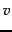in our subset of the web, we use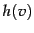to denote its hub score and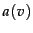its authority score. Initially, we set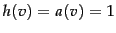for all nodes. We also denote by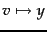the existence of a hyperlink fromto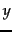. The core of the iterative algorithm is a pair of updates to the hub and authority scores of all pages given by Equation 262, which capture the intuitive notions that good hubs point to good authorities and that good authorities are pointed to by good hubs.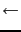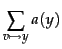(262)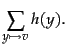(263)

Thus, the first line of Equation 262 sets the hub score of pageto the sum of the authority scores of the pages it links to. In other words, iflinks to pages with high authority scores, its hub score increases. The second line plays the reverse role; if pageis linked to by good hubs, its authority score increases.

What happens as we perform these updates iteratively, recomputing hub scores, then new authority scores based on the recomputed hub scores, and so on? Let us recast the equations Equation 262 into matrix-vector form. Let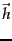and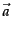denote the vectors of all hub and all authority scores respectively, for the pages in our subset of the web graph. Let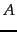denote the adjacency matrix of the subset of the web graph that we are dealing with:is a square matrix with one row and one column for each page in the subset. The entry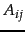is 1 if there is a hyperlink from page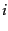to page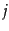, and 0 otherwise. Then, we may write Equation 262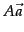(264)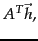(265)

where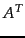denotes the transpose of the matrix. Now the right hand side of each line of Equation 264 is a vector that is the left hand side of the other line of Equation 264. Substituting these into one another, we may rewrite Equation 264 as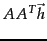(266)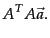(267)

Now, Equation 266 bears an uncanny resemblance to a pair of eigenvector equations (Section 18.1 ); indeed, if we replace the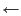symbols by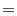symbols and introduce the (unknown) eigenvalue, the first line of Equation 266 becomes the equation for the eigenvectors of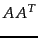, while the second becomes the equation for the eigenvectors of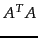: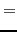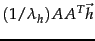(268)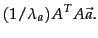(269)

Here we have used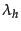to denote the eigenvalue ofand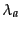to denote the eigenvalue of.

This leads to some key consequences:

1. The iterative updates in Equation 262 (or equivalently, Equation 264), if scaled by the appropriate eigenvalues, are equivalent to the power iteration method for computing the eigenvectors ofand. Provided that the principal eigenvalue ofis unique, the iteratively computed entries ofandsettle into unique steady-state values determined by the entries ofand hence the link structure of the graph.
2. In computing these eigenvector entries, we are not restricted to using the power iteration method; indeed, we could use any fast method for computing the principal eigenvector of a stochastic matrix.

The resulting computation thus takes the following form:

1. Assemble the target subset of web pages, form the graph induced by their hyperlinks and computeand.
2. Compute the principal eigenvectors ofandto form the vector of hub scoresand authority scores.
3. Output the top-scoring hubs and the top-scoring authorities.
This method of link analysis is known as HITS , which is an acronym for Hyperlink-Induced Topic Search.

Worked example. Assuming the query jaguar and double-weighting of links whose anchors contain the query word, the matrixfor Figure 21.4 is as follows: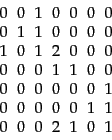(270)

The hub and authority vectors are: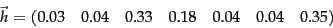(271)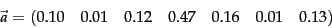(272)

Here,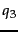is the main authority - two hubs (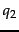and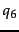) are pointing to it via highly weighted jaguar links.

End worked example.

Since the iterative updates captured the intuition of good hubs and good authorities, the high-scoring pages we output would give us good hubs and authorities from the target subset of web pages. In Section 21.3.1 we describe the remaining detail: how do we gather a target subset of web pages around a topic such as leukemia?

SubsectionsNext: Choosing the subset of Up: Link analysis Previous: Topic-specific PageRank   Contents   Index